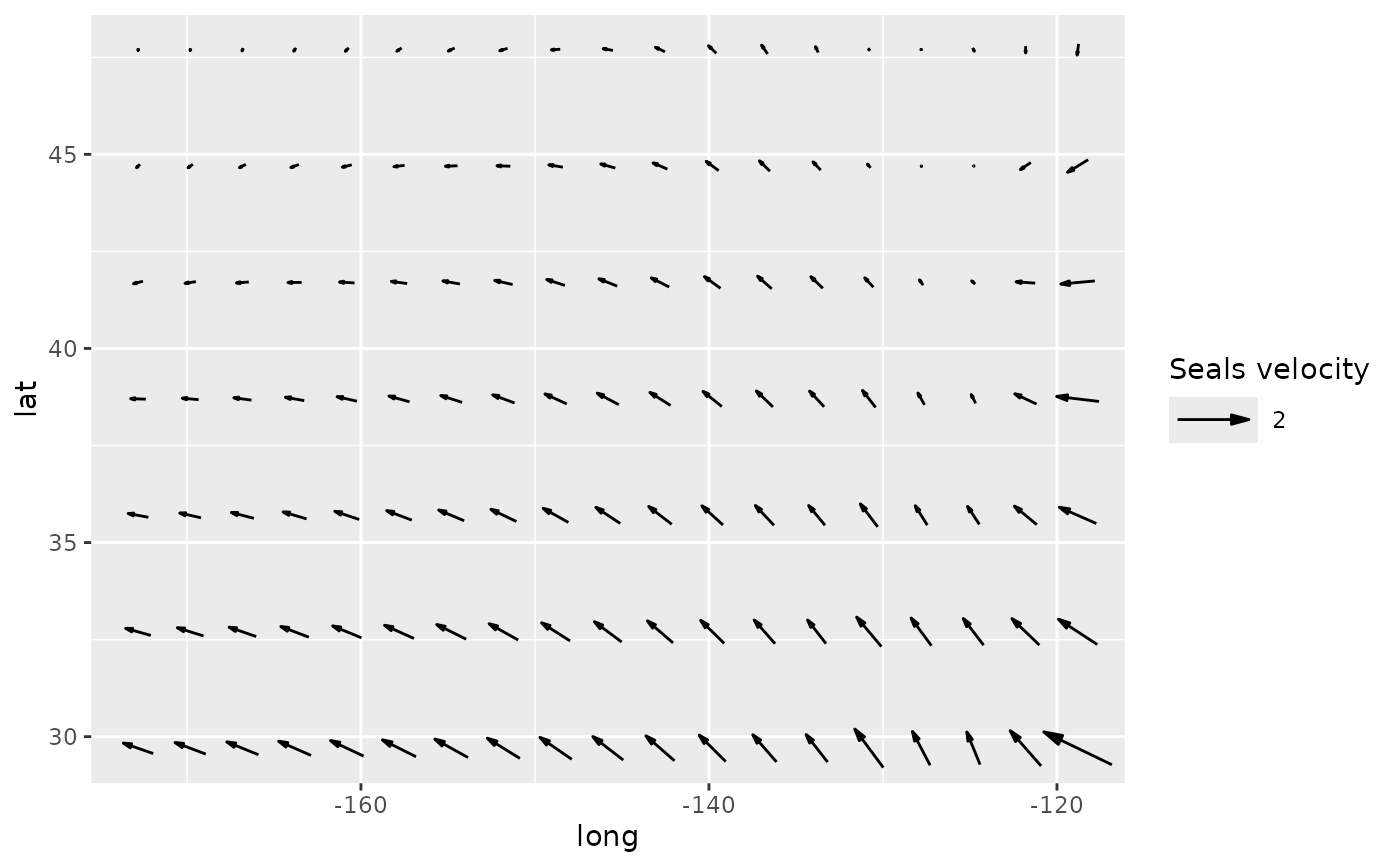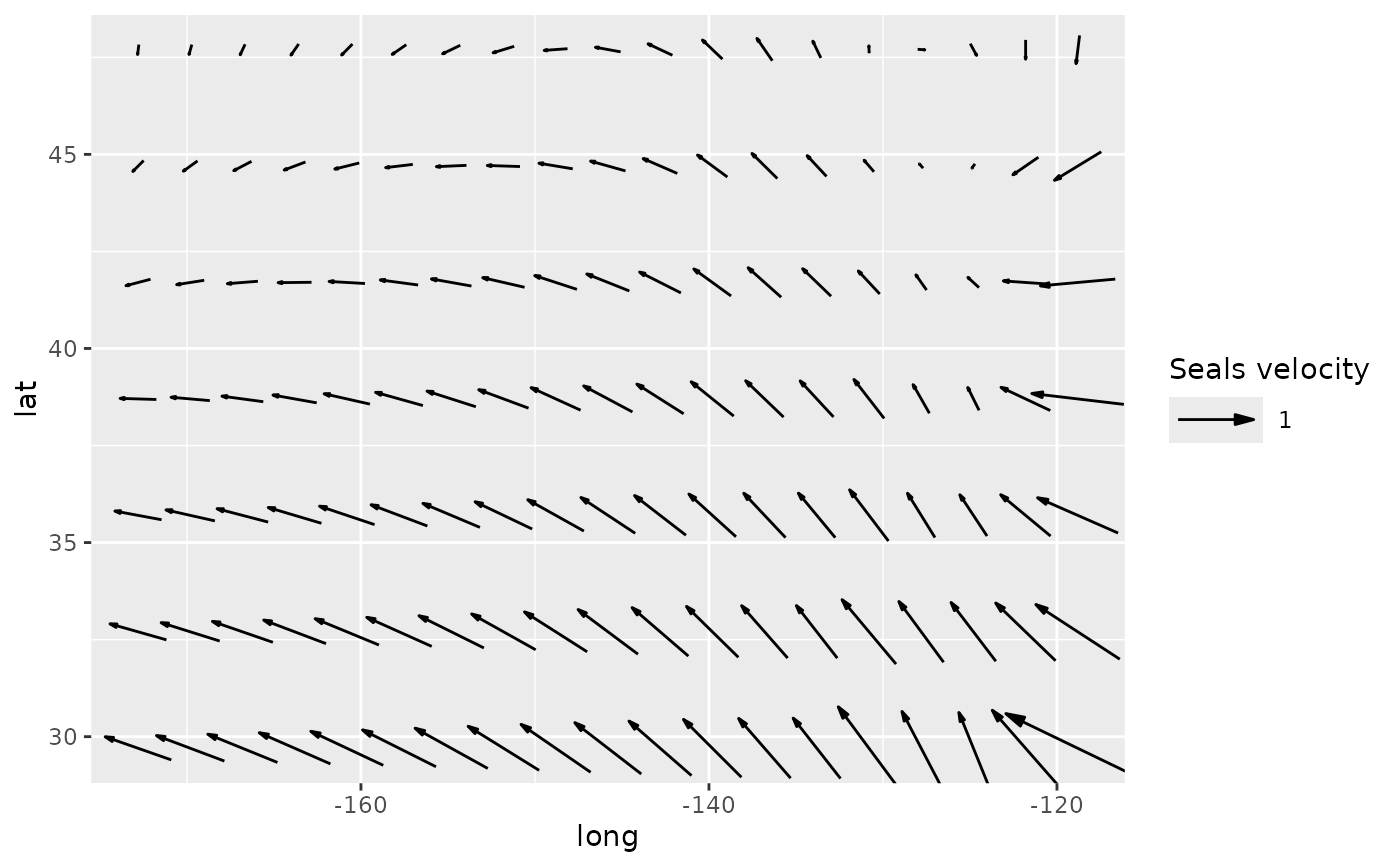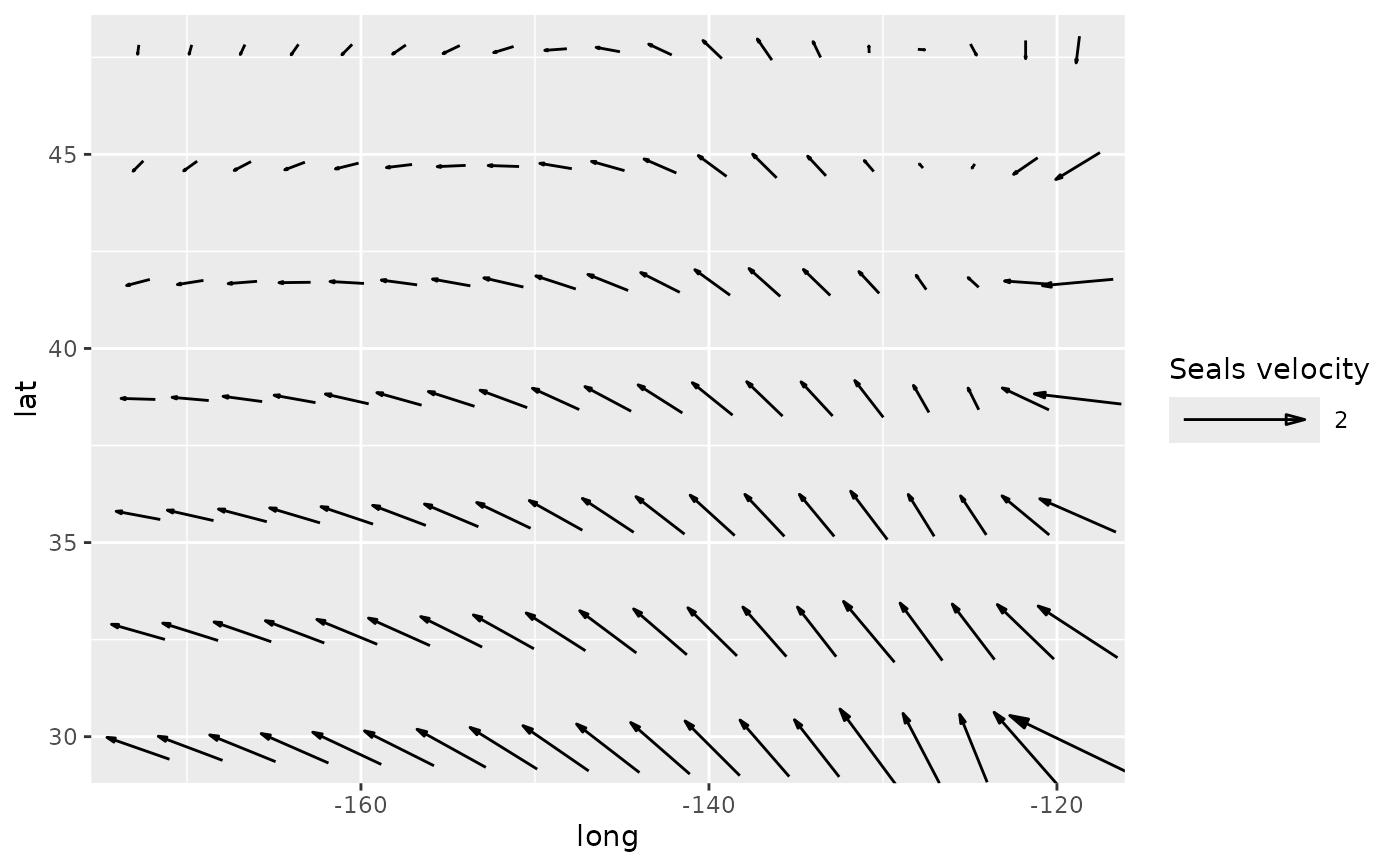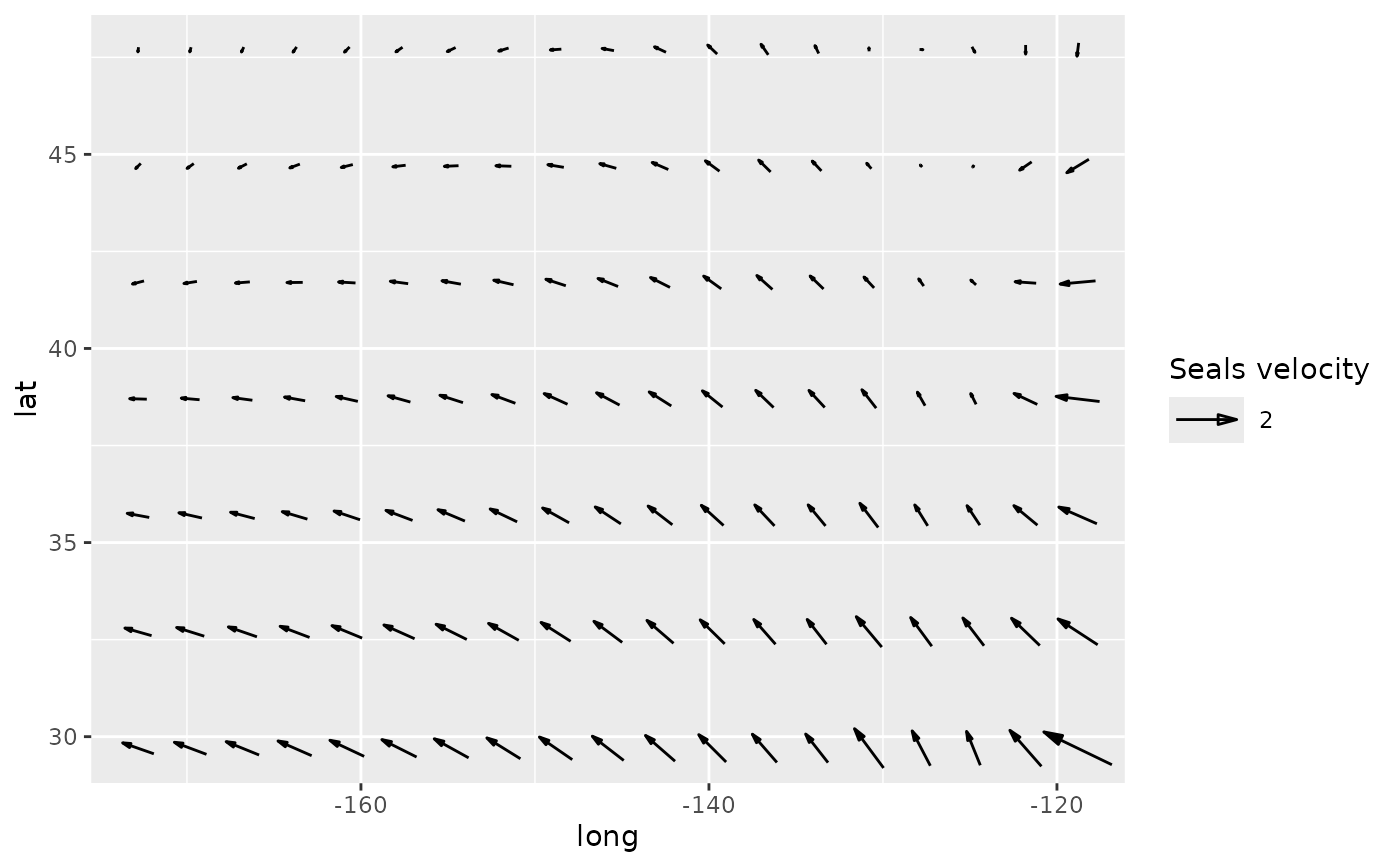Allows to control the size of the arrows in geom_arrow. Highly experimental.

scale_mag(
name = ggplot2::waiver(),
labels = ggplot2::waiver(),
max_size = 1,
default_unit = "cm",
max = ggplot2::waiver(),
guide = guide_vector(),
...
)

scale_mag_continuous(
name = ggplot2::waiver(),
labels = ggplot2::waiver(),
max_size = 1,
default_unit = "cm",
max = ggplot2::waiver(),
guide = guide_vector(),
...
)

## Arguments

name

The name of the scale. Used as the axis or legend title. If waiver(), the default, the name of the scale is taken from the first mapping used for that aesthetic. If NULL, the legend title will be omitted.

labels

One of:

• NULL for no labels

• waiver() for the default labels computed by the transformation object

• A character vector giving labels (must be same length as breaks)

• An expression vector (must be the same length as breaks). See ?plotmath for details.

• A function that takes the breaks as input and returns labels as output. Also accepts rlang lambda function notation.

max_size

size of the arrow in centimetres

default_unit

ignored

max

magnitude of the reference arrow in data units. Will be the maximum value if waiver()

guide

type of legend

...

Other arguments passed on to scale_(x|y)_continuous()

## Examples

library(ggplot2)
g <- ggplot(seals, aes(long, lat)) +
geom_vector(aes(dx = delta_long, dy = delta_lat), skip = 2)

g + scale_mag("Seals velocity")g + scale_mag("Seals velocity", max = 1)g + scale_mag("Seals velocity", max_size = 2)g + scale_mag("Seals velocity", default_unit = "mm")